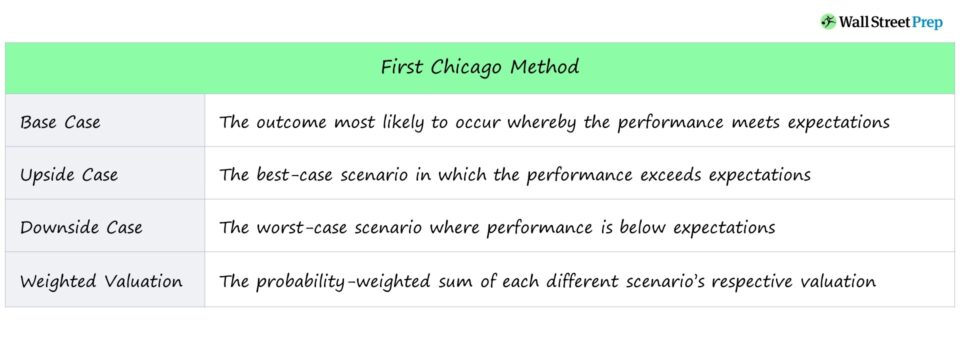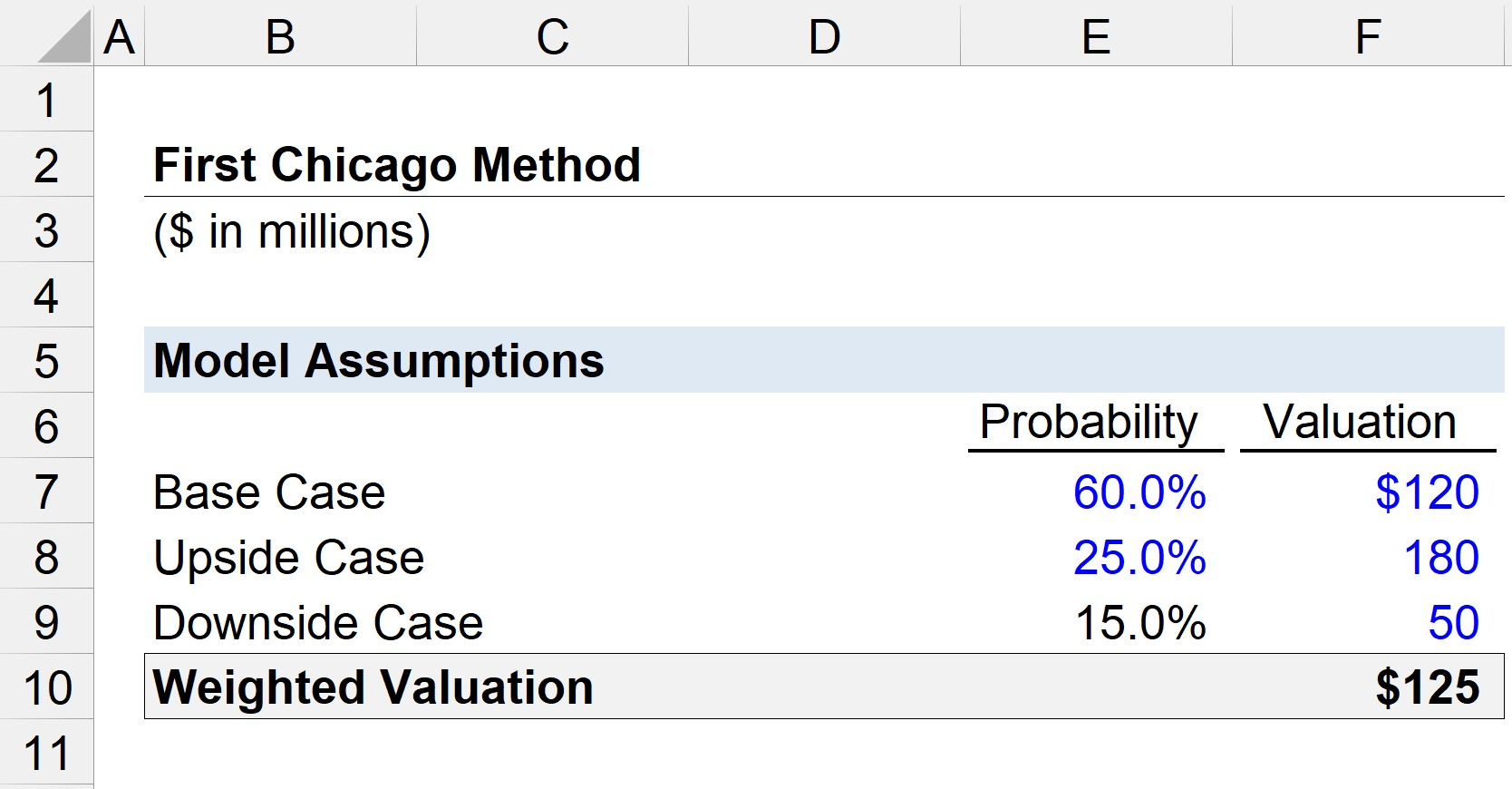Welcome to Wall Street Prep! Use code at checkout for 15% off.# First Chicago Method

Understand the First Chicago Method## First Chicago Method Overview

The First Chicago Method estimates the value of a company by taking the probability-weighted sum of three different valuation scenarios.

The method is most often used to value early-stage companies with unpredictable futures.

In practice, attempting to project the performance of high-growth companies in order to estimate the returns on an investment could prove to be difficult because of the wide range of possibilities.

Hence, the First Chicago Method is an approach to valuation in which different scenarios are probability-weighted.

## First Chicago Method – Scenario Planning

The three different scenarios consist of the following:

• Base Case → The outcome that is most likely to occur where performance meets expectations, so the highest probability weight is attached to this case.
• Upside Case → The best-case scenario in which the performance exceeds expectations, with usually the 2nd lowest probability of occurrence in most cases.
• Downside Case → The worst-case scenario where performance is below expectations, with typically the lowest probability of occurrence.

The value attributable to each case is commonly obtained from two valuation approaches:

The estimated valuation will be different in each case due to the upward or downward adjustments to the underlying assumptions that impact the valuation.

The assumptions can differ in various ways, such as the discount rate, year-over-year (YoY) growth rates, comps used in determining the exit multiple, and more.

## Base vs. Upside vs. Downside Case

The upside case and downside case are the two outcomes that are less to occur, with the latter usually being the lower likelihood of the two.

However, the reason is not that the worst-case scenario is less likely to happen, but rather that if the worst case has a higher likelihood of occurrence, it would not be worth considering an investment in the first place.

Depending on who is performing the analysis, additional cases with added contingencies could be added to the core three.

In venture investing, most investments are made with the expectation of failure, i.e. the “home runs” return the fund multiple times of their initial value and offset the losses incurred from the other failed investments.

In contrast, the base case represents the targeted performance (and returns) when integrating different cases into models for late-stage buyout investments and public equities markets.

Nevertheless, in the world of early to mid-stage investing (i.e. growth equity), the goal would be to exceed the base case.

## First Chicago Method Steps

Once the three cases are listed in a table, two other columns will be presented to the right.

1. Probability Weight (%): The likelihood that the case is expected to occur out of all potential outcomes.
2. Valuation: The DCF or VC valuation derived value that corresponds to each case.

While it should go without saying, it is still recommended to confirm that the sum of all probability weights equals 100%.

Moreover, the probability weights assigned to the upside and downside cases are usually similar.

Once the table is all set, the final step is to multiply the probability of each case by the respective valuation amount, with the sum of all the values representing the concluded implied valuation.

## First Chicago Method Pros/Cons

• Probability-Weighted Valuation of Different Outcomes
• Time-Consuming Process (Detailed Models)
• Integrates Best-Case and Down-Case Outcomes
• Subjective Probability Weight Assumptions
• Blended Approach (Flexibility) to Scenario Planning
• Unsuitable for Pre-Revenue and Seed Stage Start-Ups

## First Chicago Method Calculator – Excel Template

We’ll now move to a modeling exercise, which you can access by filling out the form below.Submitting...

## First Chicago Method Example Calculation

Suppose we are valuing a growth stage company using the First Chicago Method, with the DCF model using already completed – each with a different set of assumptions.

Our DCF model of the company approximated the valuation of the company under three different scenarios as:

• Base Case = \$120 million
• Upside Case = \$180 million
• Downside Case = \$50 million

The probability of each case was determined as the following:

• Base Case = 60%
• Upside Case = 25%
• Downside Case = 15% (1 – 85%)

Using the “SUMPRODUCT” Excel function, with the first array consisting of the probability weights while the second array consists of the valuations – we arrive at a weighted valuation of \$125 million.Step-by-Step Online Course

### Everything You Need To Master Financial Modeling

Enroll in The Premium Package: Learn Financial Statement Modeling, DCF, M&A, LBO and Comps. The same training program used at top investment banks.Inline FeedbacksLearn Financial Modeling Online

Everything you need to master financial and valuation modeling: 3-Statement Modeling, DCF, Comps, M&A and LBO.

X

The Wall Street Prep Quicklesson Series

7 Free Financial Modeling Lessons

Get instant access to video lessons taught by experienced investment bankers. Learn financial statement modeling, DCF, M&A, LBO, Comps and Excel shortcuts.